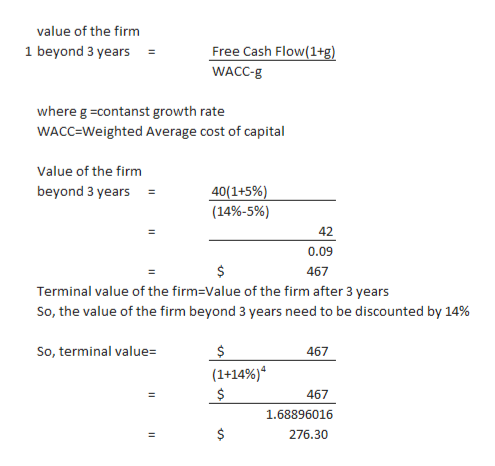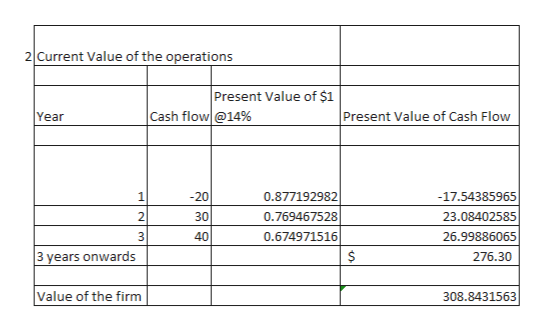Free Cash Flow ValuationDozier Corporation is a fast-growing supplier of office products. Analysts project the following free cash flows (FCFs) during the next 3 years, after which FCF is expected to grow at a constant 5% rate. Dozier's weighted average cost of capital is WACC = 14%. Year 123Free cash flow (\$ millions)-\$20\$30\$40  What is Dozier's horizon value? (Hint: Find the value of all free cash flows beyond Year 3 discounted back to Year 3.) Round your answer to two decimal places.\$ millionWhat is the current value of operations for Dozier? Do not round intermediate calculations. Round your answer to two decimal places.\$ millionSuppose Dozier has \$10 million in marketable securities, \$100 million in debt, and 10 million shares of stock. What is the intrinsic price per share? Do not round intermediate calculations. Round your answer to the nearest cent.\$

Question

Free Cash Flow Valuation

Dozier Corporation is a fast-growing supplier of office products. Analysts project the following free cash flows (FCFs) during the next 3 years, after which FCF is expected to grow at a constant 5% rate. Dozier's weighted average cost of capital is WACC = 14%.

 Year 1 2 3 Free cash flow (\$ millions) -\$20 \$30 \$40

1. What is Dozier's horizon value? (Hint: Find the value of all free cash flows beyond Year 3 discounted back to Year 3.) Round your answer to two decimal places.

\$ million

2. What is the current value of operations for Dozier? Do not round intermediate calculations. Round your answer to two decimal places.

\$ million

3. Suppose Dozier has \$10 million in marketable securities, \$100 million in debt, and 10 million shares of stock. What is the intrinsic price per share? Do not round intermediate calculations. Round your answer to the nearest cent.

\$
Step 1

The discounted cash flow method is used to determine the horizontal value, value of the firm and instrinsic value

The horizontal or terminal value is the value of the firm beyond a certain period when the growth is expected to be constant.

Step 2help_outlineImage Transcriptionclosevalue of the firm Free Cash Flow(1+g) 1 beyond 3 years = WACC-g where g -contanst growth rate WACC=Weighted Average cost of capital Value of the firm 40(1+5%) (14%-5%) beyond 3 years 42 0.09 \$ 467 Terminal value of the firm=Value of the firm after 3 years So, the value of the firm beyond 3 years need to be discounted by 14% So, terminal value 467 (1+149%) 467 1.68896016 276.30 fullscreen
Step 3help_outlineImage Transcriptionclose2 Current Value of the operations Present Value of \$1 Cash flow@14% Present Value of Cash Flow Year -20 1 0.877192982 -17.54385965 30 2 0.769467528 23.08402585 40 3 0.674971516 26.99886065 3 years onwards 276.30 Value of the firm 308.8431563 229 fullscreen

Want to see the full answer?

See Solution

Want to see this answer and more?

Our solutions are written by experts, many with advanced degrees, and available 24/7

See Solution
Tagged in